# Product to Sum and Sum to Product Formulas

The process of converting products into sums and sums into products can be a very useful tool in integration. It is also the difference in finding an easy solution versus no solution at all. The product-sum identity and the sum-product identity can be derived from the sum and difference identities.

 Sum-Product Identities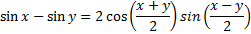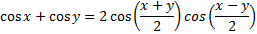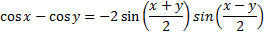Alternate forms of the sum-product identities are the product-sum identities.

 Product-Sum Identities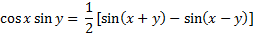Example 1: Express the product cos(3x)sin(2x) as a sum of trigonometric functions.

Step 1: Notice that the problem is the product of cosine and sine, therefore use the product- sum identityStep 2: Using substitution let x = 3x and y = 2x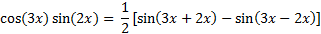Step 3: Simplify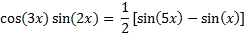Example 2: Express the sum cos(5x) + cos(7x) as a product trigonometric functions

Step 1: Notice that it is a sum of cosine and cosine, therefore use the sum-product identity:Step 2: Using substitution let x = 5x and y = 7xStep 3: Simplify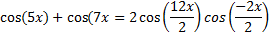Step 4: Use the even/odd function rule cos(-x) = cos (x) to replacewith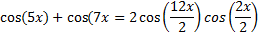Example 3: Find the exact value of sin 75° + sin 15°.

Step 1: Notice that it is a sum of sine and sine, therefore use the sum-product identity:Step 2: Using substitution let x = 75 and y = 15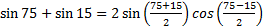Step 3: Simplify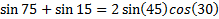Step 4: Substitute the familiar values of sin 45 =and cos 30 =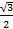into the equation and simplify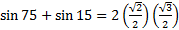Using the sum-product and the product- sum identities can make it easier to rewrite trigonometric identities in order to evaluate functions.

 Related Links: Math Trigonometry Double Angle Identities Half Angle formulas

To link to this Product to Sum and Sum to Product Formulas page, copy the following code to your site: